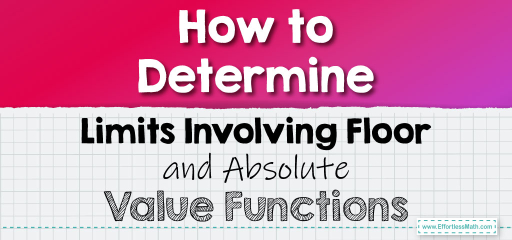# How to Determine Limits Involving Floor and Absolute Value Functions

Determining limits involving floor functions (which map a real number to the greatest integer less than or equal to it) and absolute value functions (which measure the distance of a number from zero on the real number line) can present unique challenges due to their piecewise and non-continuous nature. Let's traverse through a step-by-step guide to evaluate such limits with precision.## Step-by-step Guide to Determine Limits Involving Floor and Absolute Value Functions

Here is a step-by-step guide to determining limits involving floor and absolute value functions:

### Step 1: Understand the Behavior of Floor and Absolute Value Functions

The floor function, denoted by $$⌊x⌋$$, can cause abrupt changes in function value at integer points. Meanwhile, the absolute value function, $$∣x∣$$, introduces a kink in the graph at $$x=0$$, changing from $$x$$ to $$−x$$ as one crosses from positive to negative values. Acknowledge that these points of discontinuity and abrupt change are where we must scrutinize the limit closely.

### Step 2: Isolate the Floor and Absolute Value Components

Start by identifying the intervals on which the floor and absolute value functions are continuous and differentiable. This usually means dividing the domain of the function into segments based on integer values for the floor function and positive and negative regions for the absolute value function.

### Step 3: Piecewise Analysis

Break down the function into piecewise components. For the floor function, evaluate the limit at points just to the left and just to the right of each integer point. For the absolute value function, assess the behavior as the function approaches zero from both directions.

### Step 4: Apply Limit Definitions

Apply the definition of the limit to each piece. For the absolute value, remember that $$∣x∣=x$$ if $$x≥0$$ and $$∣x∣=−x$$ if $$x<0$$. For the floor function, consider the integer part and the fractional part of $$x$$ separately.

### Step 5: Consider One-Sided Limits

One-sided limits are essential when dealing with floor and absolute value functions. Evaluate $$lim_{x→c^−}$$​ and $$lim_{x→c^+}$$​ where $$c$$ is a point of interest (like an integer for the floor function or zero for the absolute value function). If these one-sided limits do not match, the two-sided limit does not exist.

### Step 6: Apply Limits to Each Piecewise Segment

Evaluate the limits for each segment individually. Ensure to consider the impact of the floor and absolute value operations within each interval. Use algebraic manipulation to simplify expressions where possible.

### Step 7: Reconcile Piecewise Limits

Once you have the one-sided limits, reconcile them to determine if a two-sided limit exists. If the limits from the left and right do not agree, the overall limit does not exist.

### Step 8: Check for Continuity and Differentiability

For locations where the floor or absolute value function changes, assess continuity and differentiability. The limit will exist at a point only if the function is continuous at that point.

### Step 9: Handling Combination of Functions

If the floor or absolute value function is combined with other functions, assess the impact on the limit separately. Sometimes it may be necessary to apply the limit laws in combination with the piecewise evaluation.

### Step 10: Visual Verification with Graphs

Graphing the function can provide a visual aid and verification for the analytical findings. The visual approach can be particularly helpful in identifying the behavior of the function around critical points.

## Final Word:

The evaluation of limits involving floor and absolute value functions hinges on recognizing and appropriately addressing the piecewise nature of these functions. By isolating these components and meticulously analyzing their behavior across the domain, the evaluation of such limits progresses from piecewise to holistic, resulting in a comprehensive understanding of the function’s limiting behavior.

### What people say about "How to Determine Limits Involving Floor and Absolute Value Functions - Effortless Math: We Help Students Learn to LOVE Mathematics"?

No one replied yet.

X
30% OFF

Limited time only!

Save Over 30%

SAVE $5 It was$16.99 now it is \$11.99